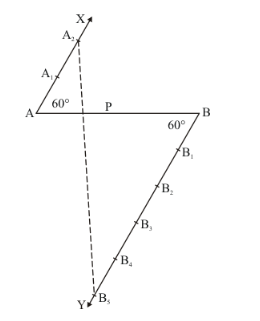# Divide a line segment of length 14 cm internally in the ratio 2 : 5.

Question:

Divide a line segment of length 14 cm internally in the ratio 2 : 5. Also, justify your construction.

Solution:

Given that

Determine a point which divides a line segment of lengthinternally in the ratio of.

We follow the following steps to construct the givenStep of construction

Step: I-First of all we draw a line segment.

Step: II- We draw a raymaking an acute anglewith.

Step: III- Draw a rayparallel to AX by making an acute angle.

Step IV- Mark of two pointsonand three pointsonin such a way that.

Step: V- Joinsand this line intersectsat a point P.

Thus, P is the point dividinginternally in the ratio ofJustification:

In $\triangle A A_{2} P$ and $\triangle B B_{5} P$, we have

$\angle A_{2} A P=\angle P B B_{5}[\angle A B Y=\angle B A X]$

And $\angle A P A_{2}=\angle B P B_{5}$ [Vertically opposite angle]

So, AA similarity criterion, we have

$\triangle A A_{2} P \approx \Delta B B_{5} P$

$\frac{A A_{2}}{B B_{5}}=\frac{A P}{B P}$

$\frac{A P}{B P}=\frac{2}{5}$